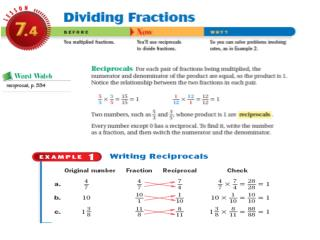DownloadDownload PresentationDividing Fractions

# Dividing Fractions

Télécharger la présentation## Dividing Fractions

- - - - - - - - - - - - - - - - - - - - - - - - - - - E N D - - - - - - - - - - - - - - - - - - - - - - - - - - -
##### Presentation Transcript

1. Dividing Fractions What is a reciprocal? How do you test a reciprocal? A reciprocal is the inverse of a fraction. If you multiply a fraction by its reciprocal they should equal 1. Ex: ¾ 4/3

2. Dividing Fractions Brainpop Dividing Fractions: KEEP CHANGE FLIP What is a reciprocal? How do you test a reciprocal?

3. Why Dividing FractionsRequires Inverting The Divisor Let's use our simple example to actually validate this strange Rule for division. If you really think about it, we are dividing a fraction by a fraction, which forms what is called a "complex fraction". It actually looks like this...

4. When working with complex fractions, what we want to do first is get rid of the denominator(1/3), so we can work this problem easier. • You may recall that any number multiplied by its reciprocal is equal to 1. And since, 1/3 x 3/1 = 1, we can use the reciprocal property of 1/3 (3/1) to make the value of the denominator equal to 1.

5. But, you might also remember that whatever we do to the denominator, we must also do to the numerator, so as not to change the overall "value". • So let's multiply both the numerator and denominator by 3/1...

6. Which gives us... Here's what happened... By multiplying the numerator and denominator by 3/1, we were then able to use the reciprocal property to eliminate the denominator. Actually, without our helpful Rule, we would have to use all of the steps above. So, the Rule for dividing fractions really saves us a lot of steps! Now that's the simplest explanation I could come up with for WHY and HOW we end up with a Rule that says we must invert the divisor!